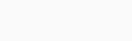# Solved – Exponential distribution: Log-Likelihood and Maximum Likelihood estimator

For a random variable with its CDF given by $$F(x)=1-exp(-lambda x),$$ and its PDF given by $$f(x)=lambda exp(-lambda x),$$ for $$x>0$$ and $$lambda >0$$.

How would I write the log-likelihood function for a random sample $$X_1,X_2,…,X_n$$ i.i.d. Exp($$lambda$$) and a maximum likelihood estimator for $$lambda$$?

I know that the exponential distribution is. I'm not quite sure where to go from there. Any help would be appreciated.

Contents

The likelihood is given as

$$L(lambda,x) = L(lambda,x_1,…,x_N) = prod_{i=1}^N f(x_i,lambda)$$

where the second identity use the IID assumption and with $$x = (x_1,…,x_N)$$. The log-likelikelihood is given as

$$l(lambda,x) := log L(lambda,x) = sum_{i=1}^N log f(x_i, lambda),$$

where $$log f(x_i,lambda) = log lambda – lambda x_i$$. This implies that

$$l(lambda,x) = sum_{i=1}^N log lambda – lambda x_i = N log lambda – lambda sum_{i=1}^N x_i.$$ Since we are interested in maximum a positive monotone transformation such as dividing with $$N$$ is fine. This gets us to

$$frac{1}{N} l(lambda , x) = log lambda – lambda bar x$$

differentiate and set to zero to get first order condition

$$frac{1}{lambda} – bar x = 0 Leftrightarrow lambda = frac{1}{bar x}$$

Small simulation in R

``lambda_0 <- 0.5 N <- 10000 x <- rexp(N, rate = lambda_0)  loglik <- function(theta)     {         ll <- N * log(theta) - theta*sum(x)         return(ll)     }  # Calculate estimate m_x <- 1/mean(x)  # Create vector for plot of loglikelihood t <- seq(0.5*m_x,1.5*m_x,length.out=100)  plot(t,loglik(t),type="l") abline(v=m_x,col="red") ``

This will genrate this plot of loglikelihood function to see maximum …Rate this post